Gaurav Gautam
93 posts
Day 101: The trick to get the inverse using the identity.

There was a lot of thinking to figure out why doing the elimination converted the Identity to the Inverse. It seems pretty simple though (although I make a bunch of mistakes with math all the time so tell me if Im wrong lol)

lets say the gaussian eliminations represented by T1, T2 and T3 convert A to I, then

T1 . T2 . T3 . A = I

or

T2 . T3 . A = T1' . I

or

T3 . A = T2' . T1' . I

or

A = T3' . T2' . T1' . I

or

A = T3' . (T1 . T2)' . I

or

A = (T1 . T2 . T3)' . I

or

A = (T1 . T2 . T3)'

or

A' = (T1 . T2 . T3)


And its with the equations like Casey Muratori wanted.

Mārtiņš Možeiko
2453 posts / 2 projects
Day 101: The trick to get the inverse using the identity.
Edited by Mārtiņš Možeiko on

That looks a lot longer evaluation than this:

T1 . T2 . T3 . A = I

T1 . T2 . T3 . A . A' = I . A'  // multiply both sides with A'

T1 . T2 . T3 . I = A'           // A . A' is I

T1 . T2 . T3 = A'

Gaurav Gautam
93 posts
Day 101: The trick to get the inverse using the identity.

This ones better

Gaurav Gautam
93 posts
Day 101: The trick to get the inverse using the identity.
Edited by Gaurav Gautam on

Hey so I also found an algebraic argument for the way the normals transform. Do you have something for this too?

Lets say we have a vector P and its normal N. Then,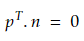And lets say we apply a transformation T to the vector P. Then, we want to find the normal such that: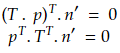So suppose there is a transformation T1 that transforms N to Nprime. Then: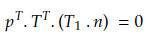So if we want to keep the dot product 0 then its sufficient that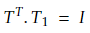Therefore we can say that if we transform a vector by the matrix T then the normal should be transformed by T1 to keep the dot product 0 and hence the transformed normal perpendicular to the transformed vector. (Incidentally, I think this means we can tranform vectors at any angle while preserving the angle like this, not just normals).

## Transformation is a rotation

Now, if the transformation T is a rotation matrix (R) then: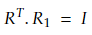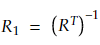And for rotation matrices we know that: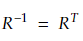So,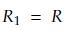Which means the normal vector n will tranform by the same rotation matrix R as the vector p.

## Transformation is scaling

For scaling we need to multiply with a diagonal matrix with the diagonal elements representing the scaling factors. So,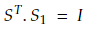Now scaling matrices will be diagonal matrices like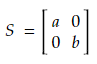and their inverse will be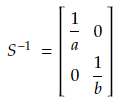and transpose of the diagonal matrix leaves it unchanged so that we have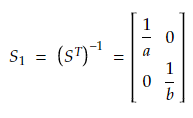## General transformation

So for a general transformation involving both rotation and scaling given by the product of transformation matrices: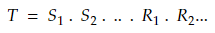The normal must be transformed as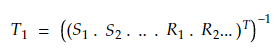or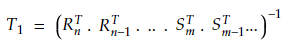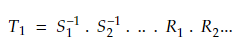So we just invert all the diagonal elements of the scaling matrices and use the rotation matrices directly for transforming normals while keeping them normal.

Robert Rebel
1 posts
Day 101: The trick to get the inverse using the identity.

To keep the dot product at zero and the converted normal perpendicular to the transformed vector, we can state that if we transform a vector by the matrix T, we must also change the normal by the matrix T1. (Incidentally, I believe this means that, in addition to normals, we may transform vectors at any angle while maintaining the angle in this manner.)

Gaurav Gautam
93 posts
Day 101: The trick to get the inverse using the identity.